# L'Hospital rule

(diff) ← Older revision | Latest revision (diff) | Newer revision → (diff)
Jump to: navigation, search

l'Hôpital's rule

A rule for removing indeterminacies of the form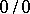orby reducing the limit of the ratio of functions to the limit of the ratio of the derivatives of the functions in question. Thus, for the case when the real-valued functionsandare defined in a punctured right neighbourhood of a pointon the number axis, l'Hospital's rule has the form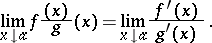(*)

Both in the case of an indeterminacy of the form, that is, whenand in the case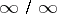, that is, when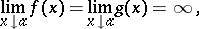l'Hospital's rule is valid under the conditions thatandare differentiable on some interval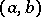,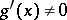for all points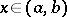, and that there is a finite or infinite limit of the ratio of the derivatives: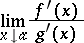(in the case of an indeterminacy of the form, this limit, if it is infinite, can only be an infinity of definite sign); then the limit of the ratio of the functions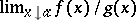exists and (*) holds. This assertion remains true, with natural changes, for the case of a left-sided and also a two-sided limit, and also when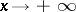or.

In a practical search for limits of ratios of functions by means of l'Hospital's rule one must sometimes apply it several times in succession.

Under the assumptions made above, the existence of a limit of the ratio of derivatives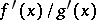is a sufficient condition for the existence of a limit of the ratioof the functions themselves, but it is not necessary.